# Identifying differential expression in multiple SAGE libraries: an overdispersed log-linear model approach

## Abstract

### Background

In testing for differential gene expression involving multiple serial analysis of gene expression (SAGE) libraries, it is critical to account for both between and within library variation. Several methods have been proposed, including the t test, t w test, and an overdispersed logistic regression approach. The merits of these tests, however, have not been fully evaluated. Questions still remain on whether further improvements can be made.

### Results

In this article, we introduce an overdispersed log-linear model approach to analyzing SAGE; we evaluate and compare its performance with three other tests: the two-sample t test, t w test and another based on overdispersed logistic linear regression. Analysis of simulated and real datasets show that both the log-linear and logistic overdispersion methods generally perform better than the t and t w tests; the log-linear method is further found to have better performance than the logistic method, showing equal or higher statistical power over a range of parameter values and with different data distributions.

### Conclusion

Overdispersed log-linear models provide an attractive and reliable framework for analyzing SAGE experiments involving multiple libraries. For convenience, the implementation of this method is available through a user-friendly web-interface available at http://www.cbcb.duke.edu/sage.

## Background

Serial analysis of gene expression (SAGE) is used to measure relative abundances of messenger RNAs (mRNAs) for a large number of genes [1, 2]. Briefly, mRNAs are extracted from biological samples and reverse-transcribed to cDNAs. The double-stranded cDNAs are then digested by a 4-cutter restriction enzyme (anchoring enzymes, usually NlaIII). After digestion, another restriction enzyme (tagging enzymes) is used to release the downstream DNA sequences at 3' of most of the anchoring enzyme restriction sites. The released sequences, usually 10–11 base pairs (bp) long, are called SAGE tags. The tags derived from many different species of mRNAs can be concatenated, cloned and sequenced. In a typical SAGE experiment, a large number of tags (often ranging from 30,000 to 100,000) are collected from each sample, with each tag representing, ideally, one gene; the tag count indicates the transcription level of the gene represented by that specific tag. A natural question of interest is whether a given tag is differentially expressed. Over the past few years, SAGE has been extensively used for expression analysis of cancer samples for identifying diagnostic or therapeutic targets [3, 4].

Most SAGE studies focus on comparing expression levels between two samples. For such two-library comparisons, several statistical methods have been proposed, such as the simulation method of Zhang et al. , the Bayesian approaches , and the normal approximation based z-test  (which is equivalent to the chi-square test ). A comparative review by Ruijter et al.  has shown that all these methods perform equally well.

The comparison between two SAGE libraries can identify biologically interesting tags (or genes). However, in many cases it is essential to conduct experiments with replicates in order to account for normal background biological variation. For experiments involving multiple SAGE libraries, between-library variation beyond the binomial sampling variation is introduced. Such between-library variation can be due to additional known factors involved in the experimental design, as well as to unknown genetic or environmental variation between observations. Indeed, major differences in gene expression exist among SAGE libraries prepared from the same tissues of different individuals . Statistical methods are needed for analyzing SAGE experiments involving multiple libraries. In the case of two-group comparisons (e.g. comparisons between a normal group and a cancer group), methods such as pooling the libraries in each group and transforming to two-library comparisons (for example, using the chi-square test), or the two-sample t- test on proportions have been proposed and discussed . The pooling approach is often problematic since it ignores gene expression variation among libraries within the same treatment group, which leads to biased estimates for the variance. The two-sample t- test on proportions, however, can be problematic as well; proportions estimated from libraries with smaller sizes are known to be more variable than those from larger libraries.

For two-group comparisons, Baggerly et al. introduced a test statistic, t w , based on a hierarchical beta-binomial model to account for both between-library and within-library variation . The t w test statistic is assumed to have an approximate t- distribution and like the t- test, the t w -test is only good for two-group comparisons. For SAGE experiments with a more general design (e.g. involving 2 or more factors), an approach based on overdispersed logistic regression has been described . Overdispersed models aim to allow for the possibility of overdispersion in the tag counts, i.e., cases where the variance in tag counts exceeds what is expected for binomial or Poisson sampling alone. Besides its flexibility in modeling multiple factors and/or continuous covariates, logistic regression compares group proportions on a logit scale (log of odds) rather than a raw scale as in the t and t w tests. Comparing groups in logistic regression (and any generalized linear model) is equivalent to testing the hypotheses of whether the coefficients β = 0. Baggerly et al.  showed evidence suggesting that "the logit scale may be more appropriate" than the original proportion scale. One drawback with overdispersed logistic regression, however, is that it can break down for cases where all the tag counts in any of groups are very small. In such cases, the deviance test rather than the t-test (on the hypothesis that the coefficient β is zero) has been proposed . Besides that a systematic evaluation of the deviance test is needed, a potential drawback with the deviance test is that it may require multiple rounds of model fitting if a model contains multiple factors or covariates. Furthermore, questions still remain on exactly when the deviance test should be used in preference to the t-test.

In this report we introduce an overdispersed log-linear model approach to analyzing SAGE which is closely related to overdispersed logistic regression but has a different mean-variance relationship assumption. We compare its performance in identifying differential expression with that of three other methods, including the t-test, t w test and overdispersed logistic regression. Analysis of simulated and real datasets show that both the log-linear and logistic overdispersion methods generally perform better than the t and t w tests. Based on simulated data, the log-linear method is found to have better performance than the logistic method, showing equal or higher statistical power over a range of parameter values and with different data distributions. The overdispersed log-linear method also appears to have better performance on the real SAGE data which we analyze; a number of cases are seen where a tag is identified by the log-linear approach and appears to be clearly differentially expressed, but which would not have been identified as significant using the logistic regression method. Overdispersed log-linear models also offer the same flexibility as logistic regression, allowing for modeling multiple factors and/or covariates. We conclude that the overdispersed log-linear models provide an attractive and reliable framework for analyzing SAGE experiments involving multiple libraries.

## Results

### Overdispersed log-linear models: a case study

Overdispersed log-linear models (see details in Methods) are very similar to overdispersed logistic models, but there are two major differences. First, overdispersed log-linear models work with logarithms of proportions (the log link) with logarithms of sample sizes m i as offsets. In contrast, overdisersped logistic models use the log of the odds (the logit link). Second, the assumption of an overdispersed log-linear model leads to derived weights used by iteratively reweighted least squares (IRLS) that depend on the means of the tag counts (i.e. the weights depend on both library sizes and tag proportions). The weights in overdispersed logistic regression, in contrast, are a function of library sizes only (see Methods).

Baggerly et al.  illustrated that the overdispersed logistic model can break down in cases where all proportions in one group are 0. Here we show that such a breakdown can also occur when the proportions in one group are small. Table 1 lists the p-values obtained from both the deviance and t tests. Note that we are testing the hypothesis that β = 0. Artificially increasing the tag counts in group 1 so that they approach the level seen in group 2 (which are held fixed), the deviance test in logistic regression and both tests (deviance and t) in the log-linear model show the expected trend of an increasing p-value (Table 1, columns 5, 6, and 7). In contrast, the p-values from the t-test in logistic regression actually decrease first and then increase (Table 1, column 4). This discrepancy between results from the t and deviance tests in the logistic model (a discrepancy not seen in the log-linear case) suggests that logistic regression can be problematic when the tag counts of all samples in one group are small.

### Simulation study

To systematically evaluate the performance of the various tests in the case of two-group comparisons, we performed a simulation study. The tests compared here are the t, t w , logit-t and log-t. For t and t w , the test is whether p A = p B, where p A and p B are the mean proportion in groups A and B respectively. The logit-t and log-t are t tests on the hypothesis of whether β = 0 in the overdispersed logistic regression and log-linear models respectively. We do not attempt to replace the t-test with the deviance test in the overdispersed logistic regression model since this requires making a possibly subjective decision on when to use one test in preference to the other.

We generated tag counts under three different distributions, choosing different tag proportions and amounts of overdispersion (Table 2). Data generated from the beta-binomial and negative binomial distributions meet the assumptions (i.e. have the mean-variance relationship structure) of the overdispersed logistic regression and log-linear models approaches, respectively. The negative binomial distribution is equivalent to the gamma-Poisson hierarchical model and is considered a robust alternative to the Poisson distribution [16, 17]. It should be noted that the t w -test is also derived under the assumption that the data is generated from a beta-binomial distribution . The range of overdispersion parameter values was chosen based on model fits from a real dataset (see section below); we used the 25, 50, and 75 percentile values of the estimated overdispersion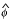from these fits. Note that the overdispersion parameter φ in the logistic model is not directly related to the φ in the log-linear model; φ values from the two models should not be compared. Given an overdispersion value φ and a group mean proportion p, the α and β values for the beta-binomial distribution are derived as α = p(1/φ - 1), and β = (1 - p)(1/φ - 1). The size parameter in the negative binomial distribution is easily derived as 1/φ. We used 5 samples (libraries) for each group, and determined the sizes of each of 10 libraries by randomly sampling from a uniform distribution over the interval between 30,000 and 90,000. This yielded library sizes of 66148, 67094, 53338, 80124, 64984, 70452, 74052, 60086, 52966 and 45377; these values were not changed over the course of the simulations. Results (not shown) from a separate run using a different set of library sizes were found to be in agreement with those shown here. A total of 5,000 sets of tag counts were generated for each combination of parameter values. The sensitivity and specificity of each of the tests were then evaluated and compared through receiver operating characteristic (ROC) curves .

The ROC curves (one for each of the four tests) shown in Figure 1 were obtained using data generated from the beta-binomial distribution (with overdispersion values φ shown on the top of the figure). Given the same false positive rate (x-axis), the overdispersion models (logistic and log-linear) clearly show improved statistical power (y-axis) compared to the two-sample t and t w tests. In contrast, when the four tests are applied to data generated from the negative binomial distribution, the overdispersed log-linear model clearly outperforms the other three tests (Figure 2). Again, the two-sample t and t w tests do not perform well in general. The figures generated using other parameter values are available [see Additional files 1 and 2]. These results suggest that for SAGE data, statistics methods based on raw proportions (as in the t and t w tests) show less power than the logistic or log-linear model approaches. The overdispersed log-linear model not only shows the best performance in cases where the data are generated in a manner consistent with its assumptions (i.e. from the negative binomial distribution), but also has competitive performance when the data come from a different distribution (here the beta-binomial). This suggests that the overdispersed log-linear model approach is more robust.

### A pancreatic cancer dataset

We further compared the four tests (t- test, t w -test, logit-t, and log-t) using an experimental SAGE data set obtained from the publicly available SAGE Genie website . To identify genes differentially expressed between the pancreatic cancer cells and normal ductal epithelium, Ryu et al.  compared the gene expression levels of five pancreatic cancer cell lines and two samples of normal pancreatic ductal epithelial cells. The library sizes and numbers of unique tags for the SAGE libraries are shown in Table 3. Note that the numbers in the table are slightly different from those described in the original paper due to the different SAGE tag processing procedures . In this analysis, we ignore tags with total counts less than 3.

We first compare the four tests by examining the overlap between the top ranking genes (top 50 and 100) identified by each test (Table 4). For the t and t w tests, the genes are ranked by the absolute value of the t (or t w ) statistics instead of by p-values (see Discussion section for details). As shown in Table 4, the results from the logit-t and log-t tests show the highest agreement (~80%); moderate agreement is observed between t w and logit-t or log-t tests (~60%) and the least agreement is seen between the t and the other three tests (~40%). The top ranking genes identified by the t-test are often those with extremely small within-group variances (data not shown). Overall, results from the t-test differ the most from the results of the other tests, while the most similar results are seen between the logit-t and log-t tests. This generally agrees with the trend seen in the simulations.

Of the top 100 genes (ranked by p-value) obtained from the logit-t and log-t tests, 82 genes are in common leaving 18 genes from each test that are not within the top 100 identified by the other test. To further examine the discrepancy between the logit-t and log-t tests, we plotted p-values obtained from both tests for these 36 remaining tags (Fig 3). It can be seen that, while tags identified by the logit-t test are also given relatively small p-values by the log-t test (all less than 0.05), those identified by the log-t test show a wide range of p-values according to the logit-t test. Table 5 lists tags which are ranked among the top 100 by the log-t test but which have p-values greater than 0.05 by the logit-t test; 4 of these were also identified by Ryu et al. . Our analysis indicates that the log-t test is relatively robust in that it not only gives reasonably small p-values to genes identified as significant by the logit-t test, but also identifies genes which would never have been considered significant by the logit-t test.

Ryu et al.  identified 49 up- and 37 down-regulated genes in cancer with the two-sample t- test and a set of rule-based methods. We compared their results with those from the log-t test (choosing the same number of top genes). Of the total of 86 genes, only 18 are in common (with 9 in each down- and up-regulated gene group). The most significant gene that is up-regulated in cancer on our list (but not in the original paper) is tag, "CTTCCAGCTA", which represents the annexin A2 gene. This gene has been reported to be up-regulated in human pancreatic carcinoma cells and primary pancreatic cancers . Another example is tag 'TTGGTGAAGG', which corresponds to the gene encoding thymosin, beta 4. This gene also has been shown to be "expressed at high levels both in tumor cell lines and in primary cultures of normal pancreas, but not in normal tissue" . A list of the top 20 genes up-regulated and the top 20 genes down-regulated in cancer based on the log-t test are listed in Table 6.

## Discussion

In this report we introduced a log-linear model with overdispersion for testing differential gene expression in SAGE. This model is closely related to the overdispersed logistic model proposed by Baggerly et al.  but with a different mean-variance relationship assumption. The differences between two models can be seen clearly in the weight (used by IRLS) associated with each observation: assuming library sizes are reasonably close, the overdispersed log-linear model tends to assign higher weights to observations in the group with the smaller mean proportion; in contrast, approximately equal weights are assigned to all the observations in the overdispersed logistic model. Although for real SAGE data the true mean-variance relationship is unknown, it has been observed that "for the higher counts data, the between-library variability is the dominant part of the variation" ; this suggests that the magnitude of the overdispersion in the group with higher counts is probably larger than that in the group with low counts so that the assumptions of the overdispersed log-linear model is probably more appropriate for SAGE data.

We also compared the model fits of the overdispersed logistic and log-linear models. Due to the introduction of the overdispersion parameter, the deviance statistic is no longer a valid basis for model fit comparison. An alternative is to use the standardized Pearson residuals, which have an asymptotic standard normal distribution . Williams  proposed the approach of plotting the standardized Pearson residuals against the predicted proportions; a problem with a model fit is indicated by a significant decrease in the variance of the standardized residuals as estimated proportions approach zero. Figure 4 shows the residual plots from the logistic and log-linear model fits for two tags (the tag counts are listed in Table 5). In the overdipersed logistic regression case (left panels of Figure 4), the variance of the standardized Pearson's residuals is seen to be much smaller in the normal group than in the cancer group. Such a difference is not evident in the overdispersed log-linear model fits (right panels of Figure 4). Although the sample size is very small in this example (only 2 in the normal group), the residual plots give further indication that log-linear models provide a better fit to SAGE data than logistic models.

From the simulation study we have shown that, besides their limitation to two-group comparison, both the t- and t w -tests, in general, are not as powerful as tests which allow for the possibility of overdispersion. We mention one specific problem that can arise with the t- and t w -tests if the number of samples in the data set is small. Note that the rank orders from the t- test and the t w test in Table 4 are based on test statistics instead of p-values. The rank orders based on p-values can be different from those based on test statistics if the residual degrees of freedom differ among tests. Both the t- test and the t w -test use the Satterthwaite approximation  for the number of degrees of freedom since the variances are assumed to be different in the two groups. An example of how this can be problematic is given by tag "AGCTGTCCCC", which has tag counts 70, 82 in the two normal samples, and 4, 1, 1, 1, 0 in the five cancer cell line samples. The differential expression is highly significant based on the logit-t (p-value 0.0003) and log-t (p-value 0.0005) tests. In contrast, if the t w -test with the Satterthwaite approximation to the degrees of freedom is used, this tag is barely significant at the 5% level (p-value 0.050). The reason is that, while the magnitude of the t w statistic for this tag is actually extremely high (|t w | = 12.01), the calculated degrees of freedom is only about 1 (which leads to low significance). The small value for the degrees of freedom arises here because the estimated variance in the cancer group is very small; the approximated degrees of freedom is then about equal to the sample size of the normal group minus 1 (here, 2-1 = 1). Cases like this occur frequently in this data set since the number of libraries (samples) in one group is very small. It is not uncommon to have small sample numbers with SAGE data.

The four methods compared in this study follow the frequentist approach of hypothesis testing, and can be broadly considered as examples of linear models. For two-group comparisons, Vencio et al.  introduced a Bayesian approach to rank tags by the Bayes Error Rate. We compared their approach with the methods based on linear models by looking at differences in gene rankings determined using the pancreatic dataset. Considering the top 100 genes identified by the different tests, the two overdispersed models show the best agreement with the Bayesian method (~70% in common); 63 genes (of the top 100) are identified by all three tests. We also evaluated the Bayesian method using the artificial data in Table 1; as the tag counts in group 1 are increased, the evidence for differential expression decreases (i.e. the Bayes Error Rate goes up), which follows the expected trend. Furthermore, if we recognize tags with p < 0.05 or E<0.1 as being significantly differentially expressed , the results from the Bayesian approach are more consistent with those from the log-linear model than from the logistic models (see Table 1). Since the evidence measures used are conceptually very different, to perform a direct comparison between "P-value"-based methods and the Bayesian approach is not straightforward. Our results, however, suggest that the Bayesian approach of Vencio is a competitive Bayesian alternative for analyzing SAGE data in the case of two-group comparisons.

The current study has not considered the issue of multiple testing problems which is still under active research [27, 28]. We note that one possible area for further improvement is to use information across genes (tags) with similar magnitude of dispersion to obtain potentially more robust and accurate overdispersion (and therefore, error) estimates. In all the methods compared here, everything is done one tag at a time, i.e., estimates of the amount of overdispersion are done for each tag individually and these can vary widely (see Figure 5). For expression data with continuous values, strategies on information sharing have been proposed  and these strategies may be adapted for discrete data such as in SAGE.

## Methods

### Data

Suppose that there are a total of n SAGE libraries in an experiment. Let m i be the size (total tag counts) of library i (i = 1..n) and r i be the tag counts for a specific tag in that library.

Also, let x i be the associated vector of explanatory variables and β the vector of coefficients. The comparison of two groups of SAGE libraries is a special case where there is only one explanatory variable associated with each observation (i.e. one factor with 2 levels).

### The two-sample t-test

The t- test proposed by Welch  was used to test whether the mean of the proportions in one group equals the mean of the other. The proportions are assumed to have unequal variances in the two groups and the degrees of freedom is calculated based on the Satterthwaite approximation as in the t w -test (see below).

### The t w -test

Baggerly et al.  introduced a beta-binomial sampling model to account for the extra-binomial variation for a simple design in which the comparison is between two groups of SAGE libraries. This is a special case of a linear model that contains one explanatory variable. Briefly, unobserved random variables P i are introduced to account for the between-library variation. For a given group, P i is assumed to have a beta distribution (α, β) with mean and variance E(P i ) = α/(α+β), and Var(P i ) = α β / [(α+β)2 (α+β+1)]. Notice that this is a special case of the form Var(P i ) = φ p i (1 - p i ) as in the overdispersed logistic model, where φ = 1/(α+β+1). Next, the group proportion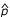is estimated by a weighted linear combination of individual proportions within the group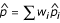, where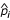= r i /m i and w i are weights associated with each individual proportion. The unbiased variance estimator ofis given as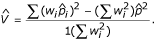To avoid having an estimated variance that is less than the binomial sampling variance, a lower-bound for the variance is also provided. All the parameters (i.e. α, β and w i ) are obtained through an iterative procedure. The same estimation procedure is applied to data from the other group. For testing whether the proportion in one group (say group A) equals the proportion in the other group (group B), a t- like statistic t w is constructed, where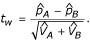The t w statistic is assumed to have a t- distribution with the degrees of freedom (df) calculated from the Satterthwaite approximation: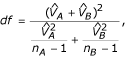where n A and n B are the number of SAGE libraries in the group A and B respectively. This test is called the t w -test here. The implementation of both the t- and t w -test can be found in .

### Overdispersed logistic regression approach

Baggerly et al.  provided a thorough description on this approach and details can be found in . Briefly, unobserved continuous random variables P i are introduced to account for the between-library variation, where the mean and variance of P i have the following forms: E(P i ) = p i ; Var(P i ) = φ p i (1 - p i ). Here φ is a nonnegative scale parameter. Conditional on P i = p i , the r i have a binomial distribution (m i , p i ). The unconditional mean and variance of r i can be shown to be E(r i ) = m i p i and Var(r i ) = m i p i (1 - p i ) [1+(m i -1) φ]. Notice that if φ is 0 (i.e. there is no between-library variation or overdispersion), the variance of r i is the usual binomial variance m i p i (1 - p i ). The estimation of coefficients β is carried out by the iteratively reweighted least-squares (IRLS) procedure, where the weights w i are 1/ [1+(m i - 1) φ]. Note that the weights w i are equal if the library sizes m i are equal.

The parameter φ is estimated by equating the goodness of fit Pearson's chi-square statistic X2 to its approximate expected value, which is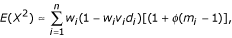where v i = m i p i (1 - p i ), and d i is the variance of the linear predictor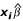. An iterative procedure is introduced to estimate φ and β, where the estimates of φ (and accordingly, the weights w i ) and β are updated at each step. Given the estimated coefficients, the testing hypothesis is whether one (or more if there are more than two groups) of the coefficients (β) is 0. For this, the t-test rather than the z-test is recommended due to the introduction of the overdispersion parameter into the model [15, 32].

The hypothesis test based on overdispersed logistic regression is called the logit-t test here. The implementation including source code can be found in . We consider overdispersion models (logistic or log-linear) only if the Pearson's chi-square statistic from the usual logistic regression (or log-linear) fit (i.e. without overdispersion) is greater than or equal to its expected value, n-p.

### Overdispersed log-linear models

This model is closely related to the overdispersed logistic regression model. One way to derive it is based on the gamma-Poisson hierarchical model assumption . Assume that an unobserved random variables θ i is distributed according to

θ i ~ Gamma(μ i , 1/φ),

where μ i = m i p i , φ >0, E(θ i ) = μ i and Var(θ i ) =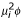. Given p i , the response variable r i is assumed to be distributed as

r i | p i ~ Poisson(μ i ).

The unconditional mean and variance of r i can be shown to be E(r i ) = μ i = m i p i and Var(r i ) = μ i (1+μ i φ). Notice that as φ decreases to 0, the variance of r i approaches the usual Poisson variance μ i (i.e. m i p i ). The same mean-variance relationship can also be derived if we assume r i has a negative- binomial distribution . The mean μ i of the response variable r i and the covariates x i are connected through the log link function,

log μ i = log(m i p i ) = x i β.

As in the overdispersed logistic regression model, the estimates of the coefficients β are obtained by the iteratively reweighted least-squares procedure, where the weights w i are 1/(1+μ i φ) . Note that, in contrast to the overdispersed logistic regression model where the weights only depend on library sizes m i , the weights in the log-linear model depend on μ i (i.e. both m i and p i ).

The hypothesis test based on overdispersed log-linear models is called the log-t test here. The R  source code and a web-interface for implementing this approach are available .

## References

1. Velculescu VE, Zhang L, Vogelstein B, Kinzler KW: Serial analysis of gene expression.[comment]. Science 1995, 270: 484–487.

2. Zhang L, Zhou W, Velculescu VE, Kern SE, Hruban RH, Hamilton SR, Vogelstein B, Kinzler KW: Gene expression profiles in normal and cancer cells. Science 1997, 276: 1268–1272. 10.1126/science.276.5316.1268

3. Riggins GJ, Strausberg RL: Genome and genetic resources from the Cancer Genome Anatomy Project. Human Molecular Genetics 2001, 10: 663–667. 10.1093/hmg/10.7.663

4. Porter D, Lahti-Domenici J, Keshaviah A, Bae YK, Argani P, Marks J, Richardson A, Cooper A, Strausberg R, Riggins GJ, Schnitt S, Gabrielson E, Gelman R, Polyak K: Molecular markers in ductal carcinoma in situ of the breast. Molecular Cancer Research: MCR 2003, 1: 362–375.

5. Audic S, Claverie JM: The significance of digital gene expression profiles. Genome Research 1997, 7: 986–995.

6. Chen H, Centola M, Altschul SF, Metzger H: Characterization of gene expression in resting and activated mast cells. Journal of Experimental Medicine 1998, 188: 1657–1668. [erratum appears in J Exp Med 1998 Dec 21;188(12):2387]. 10.1084/jem.188.9.1657

7. Lal A, Lash AE, Altschul SF, Velculescu V, Zhang L, McLendon RE, Marra MA, Prange C, Morin PJ, Polyak K, Papadopoulos N, Vogelstein B, Kinzler KW, Strausberg RL, Riggins GJ: A public database for gene expression in human cancers. Cancer Research 1999, 59: 5403–5407.

8. Kal AJ, van Zonneveld AJ, Benes V, van den Berg M, Koerkamp MG, Albermann K, Strack N, Ruijter JM, Richter A, Dujon B, Ansorge W, Tabak HF: Dynamics of gene expression revealed by comparison of serial analysis of gene expression transcript profiles from yeast grown on two different carbon sources. Molecular Biology of the Cell 1999, 10: 1859–1872.

9. Man MZ, Wang X, Wang Y: POWER_SAGE: comparing statistical tests for SAGE experiments. Bioinformatics 2000, 16: 953–959. 10.1093/bioinformatics/16.11.953

10. Ruijter JM, Van Kampen AH, Baas F: Statistical evaluation of SAGE libraries: consequences for experimental design. Physiological Genomics 2002, 11: 37–44.

11. Blackshaw S, Kuo WP, Park PJ, Tsujikawa M, Gunnersen JM, Scott HS, Boon WM, Tan SS, Cepko CL: MicroSAGE is highly representative and reproducible but reveals major differences in gene expression among samples obtained from similar tissues. Genome Biology 2003, 4: R17. 10.1186/gb-2003-4-3-r17

12. Ryu B, Jones J, Blades NJ, Parmigiani G, Hollingsworth MA, Hruban RH, Kern SE: Relationships and differentially expressed genes among pancreatic cancers examined by large-scale serial analysis of gene expression. Cancer Research 2002, 62: 819–826.

13. Baggerly KA, Deng L, Morris JS, Aldaz CM: Differential expression in SAGE: accounting for normal between-library variation. Bioinformatics 2003, 19: 1477–1483. 10.1093/bioinformatics/btg173

14. Walter-Yohrling J, Cao X, Callahan M, Weber W, Morgenbesser S, Madden SL, Wang C, Teicher BA: Identification of genes expressed in malignant cells that promote invasion. Cancer Res 2003, 63: 8939–8947.

15. Baggerly KA, Deng L, Morris JS, Aldaz CM: Overdispersed logistic regression for SAGE: modelling multiple groups and covariates. BMC Bioinformatics 2004, 5: 144. 10.1186/1471-2105-5-144

16. Casella G, Berger RL: Statistical Inferences. 2nd edition. Pacific Grove, CA: DuXBURY; 2002.

17. Gelman A, Carlin J, Stern H, Rubin D: Bayesian Data Analysis. Second edition. Boca Raton, Florida: CHAPMAN & HALL/CRC; 2004.

18. Shapiro DE: The interpretation of diagnostic tests. Stat Methods Med Res 1999, 8: 113–134. 10.1191/096228099666928387

19. SAGE Genie:[http://cgap.nci.nih.gov/SAGE]

20. Boon K, Osorio EC, Greenhut SF, Schaefer CF, Shoemaker J, Polyak K, Morin PJ, Buetow KH, Strausberg RL, De Souza SJ, Riggins GJ: An anatomy of normal and malignant gene expression.[comment]. Proceedings of the National Academy of Sciences of the United States of America 2002, 99: 11287–11292. 10.1073/pnas.152324199

21. Vishwanatha JK, Chiang Y, Kumble KD, Hollingsworth MA, Pour PM: Enhanced expression of annexin II in human pancreatic carcinoma cells and primary pancreatic cancers. Carcinogenesis 1993, 14: 2575–2579.

22. Paciucci R, Berrozpe G, Tora M, Navarro E, Garcia de Herreros A, Real FX: Isolation of tissue-type plasminogen activator, cathepsin H, and non-specific cross-reacting antigen from SK-PC-1 pancreas cancer cells using subtractive hybridization. FEBS Lett 1996, 385: 72–76. 10.1016/0014-5793(96)00352-3

23. Agresti A: Categorical Data Analysis. 2nd edition. Hoboken, New Jersey: A John Wiley & Sons, Inc., Publication; 2002.

24. Williams DA: Extra-binomial variation in logistic linear models. Applied Statistics 1982, 31: 144–148.

25. Welch BL: The generalization of 'students' problem when several different population variances are involved. Biometrika 1947, 34: 28–35.

26. Vencio RZ, Brentani H, Patrao DF, Pereira CA: Bayesian model accounting for within-class biological variability in Serial Analysis of Gene Expression (SAGE). BMC Bioinformatics 2004, 5: 119. 10.1186/1471-2105-5-119

27. Manly KF, Nettleton D, Hwang JT: Genomics, prior probability, and statistical tests of multiple hypotheses. Genome Res 2004, 14: 997–1001. 10.1101/gr.2156804

28. Choe SE, Boutros M, Michelson AM, Church GM, Halfon MS: Preferred analysis methods for Affymetrix GeneChips revealed by a wholly defined control dataset. Genome Biol 2005, 6: R16. 10.1186/gb-2005-6-2-r16

29. Kepler TB, Crosby L, Morgan KT: Normalization and analysis of DNA microarray data by self-consistency and local regression. Genome Biol 2002, 3: RESEARCH0037. 10.1186/gb-2002-3-7-research0037

30. Wright GW, Simon RM: A random variance model for detecting of differential gene expression in small microarray experiments. Bioinformatics 2003, 19: 2448–2455. 10.1093/bioinformatics/btg345

31. Cui X, Hwang JT, Qiu J, Blades NJ, Churchill GA: Improved statistical tests for differential gene expression by shrinking variance components estimates. Biostatistics 2005, 6: 59–75. 10.1093/biostatistics/kxh018

32. LIoyd CJ: Statistical Analysis of Categorical Data. New York, NY: JOhn Wiley & Sons; 1999.

33. Breslow NE: Extra-Poisson variation in log-linear models. Applied Statistics 1984, 33: 38–44.

34. R Development Core Team: R: A language and environment for statistical computing. R Foundation for Statistical Computing, Vienna, Austria; 2003.

35. The website of overdispersed log-linear models for SAGE:[http://dulci.biostat.duke.edu/sage]

## Acknowledgements

The authors would like to thank anonymous reviewers for several constructive comments. We thank Gregory Riggins for introducing us to SAGE. We gratefully acknowledge the financial support of the NIH through the Duke University Center for Translational Research (5 P30 AI051445-03) and through the Southeast Regional Center of Excellence in Biodefense and Emerging Infections (U54 AI057157-02); and of the NSF through a grant to our collaborator David Bird (NCSU; DBI 0077503) as well as the Duke Center for Bioinformatics and Computational Biology for support through a postdoctoral fellowship to JL.

## Author information

Authors

### Corresponding author

Correspondence to Thomas B Kepler.

### Authors' contributions

JL developed the method. JL and JKT carried out the simulation and data analysis. JKT and JL set up the web interface for implementing this approach. TBK supervised the study, and assisted with the methodology. All authors contributed to the writing, read and approved the final manuscript.

## Electronic supplementary material

### 12859_2005_490_MOESM1_ESM.gz

Additional File 1: This gzipped tar file contains figures showing the receiver operating characteristic curves (ROC) for the four tests applied to datasets generated from the beta-binomial distribution with various magnitudes of overdispersion(φ) and mean proportions. For example, the file 2_8e-06_0.0002.png shows the ROC curves when p B = 2p A , φ = 8e-06 and p A = 0.0002. (GZ 119 KB)

### 12859_2005_490_MOESM2_ESM.gz

Additional File 2: Similar to the file above, this file contains figures of ROC curves but with data generated from the negative binomial distribution. (GZ 142 KB)

## Authors’ original submitted files for images

Below are the links to the authors’ original submitted files for images.

## Rights and permissions

Reprints and Permissions

Lu, J., Tomfohr, J.K. & Kepler, T.B. Identifying differential expression in multiple SAGE libraries: an overdispersed log-linear model approach. BMC Bioinformatics 6, 165 (2005). https://doi.org/10.1186/1471-2105-6-165

• Accepted:

• Published:

• DOI: https://doi.org/10.1186/1471-2105-6-165

### Keywords

• Library Size
• Deviance Test
• Iteratively Reweighted Little Square
• Overdispersion Parameter
• Satterthwaite Approximation